+
Writing Equivalent Equations
Solving Linear Equations and Inequalities
0
of 0 possible points

# Writing Equivalent Equations

Author: Sophia Tutorial
##### Description:

Determine if equations are equivalent by solving each equation and comparing their solution.

(more)

Sophia’s self-paced online courses are a great way to save time and money as you earn credits eligible for transfer to many different colleges and universities.*

No credit card required

46 Sophia partners guarantee credit transfer.

299 Institutions have accepted or given pre-approval for credit transfer.

* The American Council on Education's College Credit Recommendation Service (ACE Credit®) has evaluated and recommended college credit for 33 of Sophia’s online courses. Many different colleges and universities consider ACE CREDIT recommendations in determining the applicability to their course and degree programs.

Tutorial
what's covered
1. Equivalent Equations
2. Determining if Two Equations are Equivalent

# 1. Equivalent Equations

In mathematics, we work with equivalent equations all the time. Think about the process for solving a multi-step equation. We might start with something such as 5x + 3 = 23 Using inverse operations, we create a series of equivalent equations in order to find a value for x.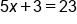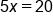An equivalent equation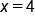An equivalent equation

The equations above are all considered equivalent equations, because they have the same solution. In each equation, the solution is x = 4.

# 2. Determining if Two Equations are Equivalent

In order to determine if two equations are equivalent, we will solve each equation, and then compare their solutions. If their solutions are the same, we can say the equations are equivalent. If the solutions are not the same, we know that the equations are not equivalent.

Determine if the equations below are equivalent: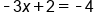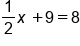Solve each equation separately: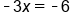Subtract 6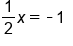Subtract 9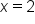Divide by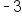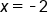Multiply by 2

The solutions to our equations are x = 2 and x = –2. Since the solutions are not the same, the two equations are not equivalent.

summary
We can define equivalent equations as equations that have the same solution or solution set. To determine if two equations are equivalent to each other, you just need to solve each equation and then determine if their solutions are the same.

Rating